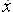Go to contentThe displays below shows how the derivative of the exponential function e^x is found by using "differentiation from first principles". This version uses the \delta y, \delta x method, where \delta y means "a small increase in y" and \delta x means "a small increase in x".

## Summary/Background

The above display makes use of an important factor about the exponential function, namely that, as \delta x \to 0 so \displaystyle \frac{e^{\delta x} - 1}{\delta x} \to 1 .You can get a better display of the maths by downloading special TeX fonts from jsMath. In the meantime, we will do the best we can with the fonts you have, but it may not be pretty and some equations may not be rendered correctly.

## Glossary

### derivative

rate of change, dy/dx, f'(x),, Dx.

### exponential function

A function having variables as exponents.

### function

A rule that connects one value in one set with one and only one value in another set.

### union

The union of two sets A and B is the set containing all the elements of A and B.

Full Glossary List

## This question appears in the following syllabi:

SyllabusModuleSectionTopicExam Year
AP Calculus AB (USA)3Differentiationex-
AP Calculus BC (USA)3Differentiationex-
AQA A-Level (UK - Pre-2017)C3Differentiationex-
AQA A2 Maths 2017Pure MathsDifferentiationDifferentiating Exponentials-
AQA AS/A2 Maths 2017Pure MathsDifferentiationDifferentiating Exponentials-
CCEA A-Level (NI)C3Differentiationex-
CIE A-Level (UK)P2Differentiationex-
Edexcel A-Level (UK - Pre-2017)C3Differentiationex-
Edexcel A2 Maths 2017Pure MathsDifferentiationDifferentiating Exponentials-
Edexcel AS/A2 Maths 2017Pure MathsDifferentiationDifferentiating Exponentials-
I.B. Higher Level6Differentiationex-
I.B. Standard Level6Differentiationex-
Methods (UK)M8Differentiationex-
OCR A-Level (UK - Pre-2017)C3Differentiationex-
OCR A2 Maths 2017Pure MathsDifferentiation FundamentalsDifferentiating Exponentials-
OCR MEI A2 Maths 2017Pure MathsDifferentiation FundamentalsDifferentiating Exponentials-
OCR-MEI A-Level (UK - Pre-2017)C3Differentiationex-
Pre-U A-Level (UK)4Differentiationex-